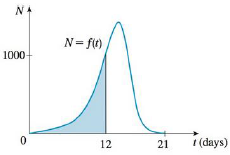Chapter 5.1, Problem 19E### Single Variable Calculus: Early Tr...

8th Edition
James Stewart
ISBN: 9781305270343

#### Solutions

Chapter
Section### Single Variable Calculus: Early Tr...

8th Edition
James Stewart
ISBN: 9781305270343
Textbook Problem

# In someone infected with measles, the virus level N (measured in number of infected cells per mL of blood plasma) reaches a peak density at about t = 12 days (when a rash appears) and then decreases fairly rapidly as a result of immune response. The area under the graph of N(t) from t = 0 to t = 12 (as shown in the figure) is equal to the total amount of infection needed to develop symptoms (measured in density of infected cells × time). The function N has been modeled by the function f ( t ) = − t ( t − 21 ) ( t + 1 ) Use this model with six subintervals and their midpoints to estimate the total amount of infection needed to develop symptoms of measles.Source: J. M. Heffernan et al., “An In-Host Model of Acute Infection: Measles as a Case Study,” Theoretical Population Biology 73 (2006): 134–47.

To determine

The total amount of infection needed to develop symptoms of measles.

Explanation

Given information:

The virus level reaches the peak density at t=12days.

The function N is modeled by the function f(t)=t(t21)(t+21).

The subintervals is n=6.

Calculation:

Draw the graph of N(t) as shown in Figure 1.

Find the width (Δt) using the relation:

Δt=ban (1)

Here, the upper limit is b, the lower limit is a, and the number of rectangles is n.

Substitute 12 for b, 0 for a, and 6 for n in Equation (2).

Δt=1206=2

The intervals are 0, 2 days, 4 days, 6 days, 8 days, 10 days, and 12 days.

The midpoints of the intervals are 1 day, 3 days, 5 days, 7 days, 9 days, and 11 days.

The expression to find the total amount of infection needed to develop symptoms of measles (Mn) is shown below:

Mn=f(t1)Δt+f(t2)Δt+...+f(tn)Δt (2)

Rearrange Equation (2) for the n=6 and mid points of the intervals as shown below:

M6=f(1)Δt+f(3)Δt+f(5)Δt+f(7)Δt+f(9)Δt+f(11)Δt (3)

Refer Figure 1

### Still sussing out bartleby?

Check out a sample textbook solution.

See a sample solution

#### The Solution to Your Study Problems

Bartleby provides explanations to thousands of textbook problems written by our experts, many with advanced degrees!

Get Started

#### Symmetry Test the equation for symmetry. 105. y = x4 + x2

Precalculus: Mathematics for Calculus (Standalone Book)

#### In Exercises 107-120, factor each expression completely. 117. 9x2 16y2

Applied Calculus for the Managerial, Life, and Social Sciences: A Brief Approach

#### For f(x) = 3x + 1 and g(x) = x, ?

Study Guide for Stewart's Single Variable Calculus: Early Transcendentals, 8th